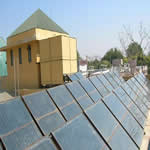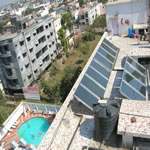# SHREYANS ENERGY PVT. LTD.

### GallerySolar Hot water SystemSolar Hot Water SystemSolar Hot Water System

CASE STUDY

Solar Hot Water System for Five Star Hotel

Installation and commissioning

Nos. of Rooms in the hotel                                                                     -  66

Solar hot water system capacity
Calculated @ 55 ltrs. Per room                                                             - 4000 LPD

ALTERNATE  I

A) Electrical Geyser Installation in each room
Cost of geyser                                                                                 -Rs. 4000=00
Cost of cable, switches, MCB etc                                                     -Rs. 1500=00
Total cost of installation                                                                -Rs. 3,63,000=00

B) Operation Cost
Monthly demand charges 198 KW@ 180 per KW                                -Rs. 35,640=00
Annual Demand charges 35640 X 12                                                  -Rs. 4,27,680=00
Energy charges for 186 units/day X 200 days
@  Rs. 5.0 Per KW                                                                          -RS 1,86,000=00
Annual operating cost.                                                                  -Rs. 6,13,680=00

ALTERNATE  II
A) Solar hot water system installation
Installation of solar hot water system with an arrangement
of instant hot water availability in all the 66 rooms.

Cost of solar hot water system                                                           -Rs. 6,50,000=00
Cost of piping                                                                                   -Rs. 3,00,000=00
Total cost of installation                                                                 -Rs. 9,53,000=00

B) Operating cost
Monthly demand charges on backup electrical
heating system           12 KW @ 180per month X 12                             -Rs. 25,920=00
Energy Charges for 50 days of cloudy days in a year                             -Rs. 72,000=00
Electrical energy input to circulating water in a year                               -Rs. 36,000=00
Annual Operating cost.                                                                 -  Rs. 1,33,920=00

 Cost Solar hot water system (Rs.) Electrical Geyser (Rs.) Capital cost (with out interest ) 9,50,000=00 3,96,000=00 Operating cost (with out interest) 1,33,920=00 6,13,680=00 Total cost after 1st year of operation 10,83,920=00 10,09680=00

Conclusion : Solar hot water system shall be cheaper by Rs. 4,79,760=00(613680-133920) from 2nd year onwards in comparison to electrical geyser installation.